## How to use tensor products

### Quick description

The tensor product is a way to encapsulate the notion of bilinearity, and can be thought of as a multiplication of two vector spaces.

### Prerequisites

Linear algebra.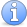Incomplete This article is incomplete. Many more examples needed, demonstrating different ways in which tensor products are used. And more general discussion of the different uses is needed too.

### General discussion

The dimension of a tensor product of two vector spaces is precisely the product of their dimensions, so when one wishes to show that a certain vector space is finite dimensional, one can try to show that it is a subspace of a tensor product (or an image of a tensor product) of two finite dimensional vector spaces.

### Example 1

Fix a field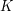. Some notation: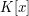is the polynomial ring in one variable,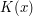is the field of rational functions,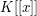is the ring of formal power series, and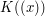is the field of formal Laurent series.

A power series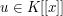is said to be D-finite if it satisfies a linear differential equation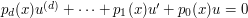for some polynomials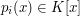with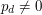, and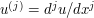. Let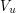denote the-subspace ofspanned by the derivatives of. Then the property of being D-finite can be seen to be equivalent to requiring that the subspaceis finite dimensional over. From this, it is easy to see that the sum of two D-finite generating functions is also D-finite since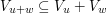. But what about the product of two D-finite generating functions?

We can define a map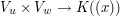by multiplication: the pair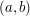simply goes to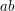. The subspace spanned by the image of this will contain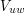by the Leibniz rule for taking the derivative of a product. But this map is not linear, so we cannot say much about the dimension of this span. However, it is bilinear, and hence we have an associated linear map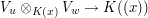whose image is precisely the span of the image of the bilinear map, and we see then that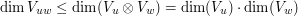, sois also D-finite.

### Example 2

This finite dimensionality argument is used when proving a basic result about affine algebraic groups over fields, namely that they admit a faithful linear representation (and thus are rightfully called linear algebraic groups).

An affine algebraic group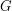is of the form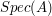where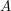is a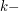algebra of finite type endowed with a comultiplication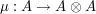. Constructing a faithful linear representation ofboils down to finding a surjection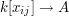where the polynomial ring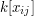is endowed with the usual Hopf algebra structure. This is done by choosing carefully a finite set of generators of thealgebrain such a way that the spanned finite dimensionalvector space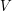satisfies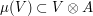and writing down the coefficients. See e.g Borel's book Linear Algebraic Book, sections I.1.9 and I.1.10

### Can the notation be

Can the notation be explained? Isdifferent fromdifferent from?

### Clarification

Anonymous:
Usually, all those are different algebraic structures:represents the ring of polynomials on one variable () with coefficients on, i.e., \$K[x]=\{\sum_{i=0}^n k_i

## Post new comment

(Note: commenting is not possible on this snapshot.)

Before posting from this form, please consider whether it would be more appropriate to make an inline comment using the Turn commenting on link near the bottom of the window. (Simply click the link, move the cursor over the article, and click on the piece of text on which you want to comment.)